# Analysis of Exponential Fourier Series

## Fourier Series at a Glance

A continuous time signal x(t) is said to be periodic if there is a positive non-zero value of T for which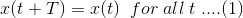As we know any periodic signal can be classified into harmonically related sinusoids or complex exponential, provided it satisfies the Dirichlet’s Conditions. This decomposed representation is called FOURIER SERIES.
Two type of Fourier Series representation are there. Both are equivalent to each other.

• Exponential Fourier Series
• Trigonometric Fourier Series

Both representations give the same result. Depending upon the type of signal, we choose any of the representation according to our convenience.

## Exponential Fourier Series

A periodic signal is analyzed in terms of Exponential Fourier Series in the following three stages:

1. Representation of Periodic Signal.
2. Amplitude and Phase Spectra of a Periodic Signal.
3. Power Content of a Periodic Signal.

### Representation of Periodic Signal

A periodic signal in Fourier Series may be represented in two different time domains:

1. Continuous Time Domain.
2. Discrete Time Domain.

#### Continuous Time Domain

The complex Exponential Fourier Series representation of a periodic signal x(t) with fundamental period To is given byWhere, C is known as the Complex Fourier Coefficient and is given by,Where ∫0T0, denotes the integral over any one period and, 0 to T0 or –T0/2 to T0/2 are the limits commonly used for the integration.
The equation (3) can be derived be multiplying both sides of equation (2) by e(-jlω0t) and integrate over a time period both sides.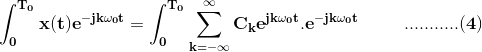On interchanging the order of summation and integration on R.H.S., we get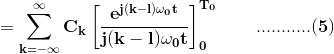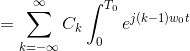When, k≠l, the right hand side of (5) evaluated at the lower and upper limit yields zero. On the other hand, if k=l, we have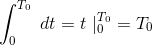Consequently equation (4) reduces to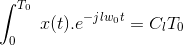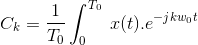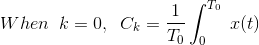which indicates average value of x(t) over a period.
When x (t) is real,Where, * indicates conjugate

#### Discrete Time Domain

Fourier representation in discrete is very much similar to Fourier representation of periodic signal of continuous time domain.
The discrete Fourier series representation of a periodic sequence x[n] with fundamental period No is given byWhere, Ck, are the Fourier coefficients and are given by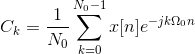This can be derived in the same way as we derived it in continuous time domain.

### Amplitude and Phase Spectra of a Periodic Signal

We can express Complex Fourier Coefficient, Ck as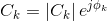A plot of |Ck| versus the angular frequency w is called the amplitude spectrum of the periodic signal x(t), and a plot of Фk, versus w is called the phase spectrum of x(t). Since the index k assumes only integers, the amplitude and phase spectra are not continuous curves but appear only at the discrete frequencies kω0, they are therefore referred to as discrete frequency spectra or line spectra.
For a real periodic signal x (t) we have C-k = Ck*. Thus,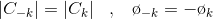Hence, the amplitude spectrum is an even function of ω, and the phase spectrum is an odd function of 0 for a real periodic signal.

### Power Content of a Periodic Signal

Average Power Content of a Periodic Signal is given byIf x (t) is represented by the complex exponential Fourier Series, then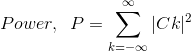This equation is known as Parseval’s identity or Parseval’s Theorem.

Want To Learn Faster? 🎓
Get electrical articles delivered to your inbox every week.
No credit card required—it’s 100% free.